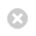Close

Institute of Education

Home# Cornerstone Maths

Cornerstone Maths is a set of targeted curriculum units of work for key stage 3 maths that help pupils achieve deep learning of the most difficult mathematics concepts through guided explorations and activities that use dynamic technology to foster reasoning and collaboration.

The three curriculum units address:

• Algebraic patterns and expressions: the concept of an algebraic variable; describing repeating patterns using algebra; and justifying algebraic equivalence.
• Linear functions: controlling and describing linear motion using animations, graphs, tables and equations; exploring y=mx+c as a model for linear motion and describing piece-wise linear functions.
• Geometric similarity: identifying the variants and invariants in shapes that are mathematically similar and recognising the one-to-one geometric correspondence of sides and vertices within mathematically similar polygons.

Professional development (PD) is another key facet of the project. Schools currently using Cornerstone Maths report school-wide change in the way teachers approach mathematics education for students of different ages and abilities.